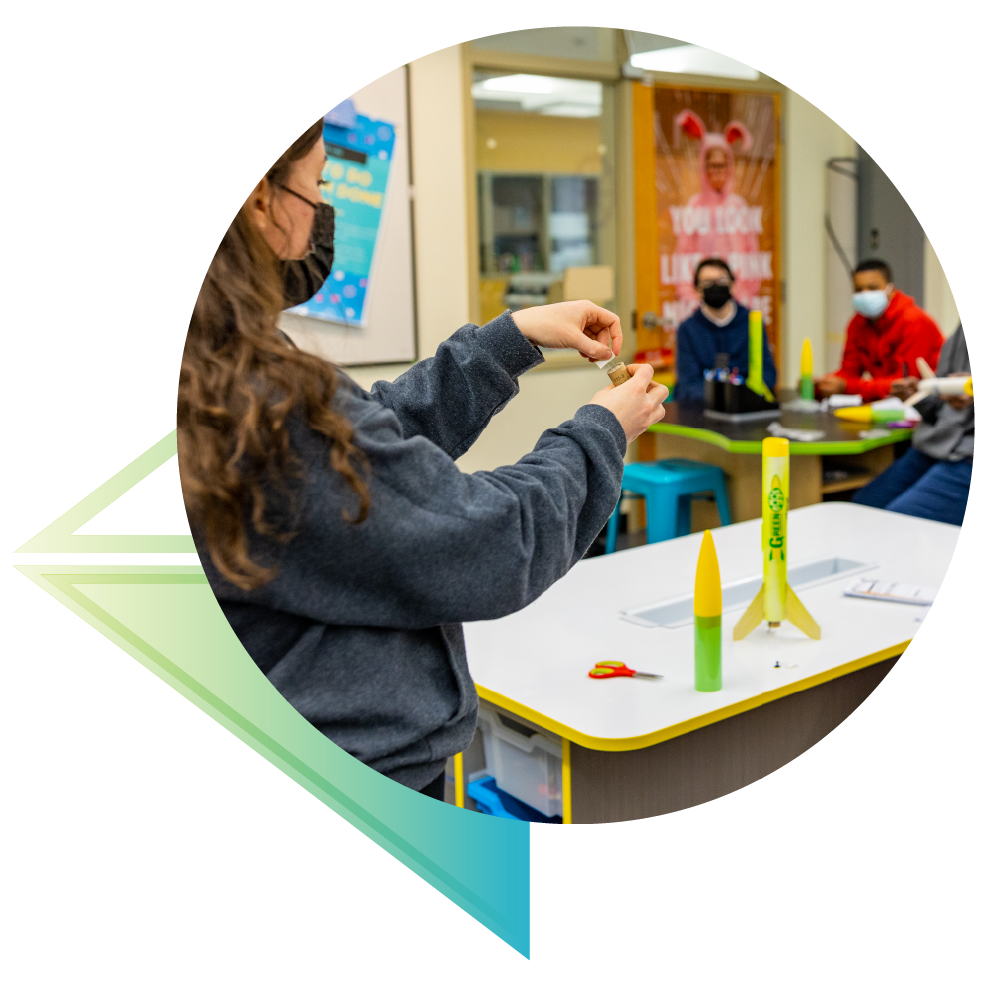# An Occasion for An Equation - Unit Plan

## An Occasion for An Equation - Unit Plan

View full details

## Grades 6-8 | 10 (45 min) Classes

In this unit, students will use algebraic equations and expressions to make sense of what effect different masses have on the velocity and acceleration of different model rockets. They will answer the question, “How are algebraic equations used to calculate model rocket acceleration?” and will learn how to calculate altitude, velocity, and acceleration using basic algebraic equations of motion.

For their final assessment, students will create a bar graph of the average acceleration for each of the three rockets (Alpha, Generic E2X, and Viking) and reflect on the effect of the mass of the rocket on acceleration.

## Common Core Standards - Math

### CCSS.MATH.CONTENT.6.EE.A.1

Write and evaluate numerical expressions involving whole-number exponents.

### CCSS.MATH.CONTENT.6.EE.A.2

Write, read, and evaluate expressions in which letters stand for numbers.

### CCSS.MATH.CONTENT.6.EE.A.2.A

Write expressions that record operations with numbers and with letters standing for numbers.

### CCSS.MATH.CONTENT.6.EE.A.2.B

Identify parts of an expression using mathematical terms (sum, term, product, factor, quotient, coefficient); view one or more parts of an expression as a single entity.

### CCSS.MATH.CONTENT.6.EE.A.2.C

Evaluate expressions at specific values of their variables. Include expressions that arise from formulas used in real-world problems. Perform arithmetic operations, including those involving whole-number exponents, in the conventional order when there are no parentheses to specify a particular order (Order of Operations).

### CCSS.MATH.CONTENT.6.EE.B.5

Understand solving an equation or inequality as a process of answering a question: which values from a specified set, if any, make the equation or inequality true? Use substitution to determine whether a given number in a specified set makes an equation or inequality true.

### CCSS.MATH.CONTENT.6.EE.B.6

Use variables to represent numbers and write expressions when solving a real-world or mathematical problem; understand that a variable can represent an unknown number, or, depending on the purpose at hand, any number in a specified set.

### CCSS.MATH.CONTENT.6.EE.B.7

Solve real-world and mathematical problems by writing and solving equations of the form x + p = q and px = q for cases in which p, q and x are all nonnegative rational numbers.

### CCSS.MATH.CONTENT.6.EE.C.9

Use variables to represent two quantities in a real-world problem that change in relationship to one another; write an equation to express one quantity, thought of as the dependent variable, in terms of the other quantity, thought of as the independent variable. Analyze the relationship between the dependent and independent variables using graphs and tables and relate these to the equation.

### CCSS.MATH.CONTENT.7.EE.A.1

Apply properties of operations as strategies to add, subtract, factor, and expand linear expressions with rational coefficients.

### CCSS.MATH.CONTENT.7.EE.B.3

Solve multi-step real-life and mathematical problems posed with positive and negative rational numbers in any form (whole numbers, fractions, and decimals), using tools strategically. Apply properties of operations to calculate with numbers in any form; convert between forms as appropriate; and assess the reasonableness of answers using mental computation and estimation strategies.

### CCSS.MATH.CONTENT.7.EE.B.4

Use variables to represent quantities in a real-world or mathematical problem and construct simple equations and inequalities to solve problems by reasoning about the quantities.

### CCSS.MATH.CONTENT.7.EE.B.4.A

Solve word problems leading to equations of the form px + q = r and p(x + q) = r, where p, q, and r are specific rational numbers. Solve equations of these forms fluently. Compare an algebraic solution to an arithmetic solution, identifying the sequence of the operations used in each approach.

### CCSS.MATH.CONTENT.8.EE.B.5

Graph proportional relationships, interpreting the unit rate as the slope of the graph. Compare two different proportional relationships represented in different ways.

### CCSS.MATH.CONTENT.8.EE.C.7

Solve linear equations in one variable.

## Common Core Standards - ELA

### CCSS.ELA-LITERACY.RST.6-8.3

Follow precisely a multistep procedure when carrying out experiments, taking measurements, or performing technical tasks.

## Building Supplies## Live, Online Training and 1:1 Support!

Estes Education has been a leader in STEM education for over 60 years. We know that STEM is a vital interdisciplinary topic that requires hands-on and inclusive learning. Explore these supporting materials to take learning to new heights!

Should you have need, you can meet with a member of Education team for FREE, 1:1 online support to learn rocketry basics, how to apply our curriculum, and discover unique teaching strategies. Our team is eager to answer your questions!## Learn About Model Rocket Safety!

Not sure how to safely launch a rocket with your group? Head over to our dedicated Safety instructions page for videos, support, and more!